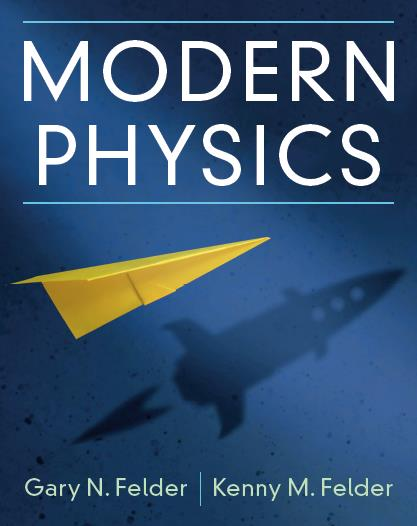# Modern Physics:Contents

## by Gary N. Felder and Kenny M. Felder

Below is a list of the 14 chapters and 10 appendices in the book. Professors can contact Cambridge University Press to request an evaluation copy.

Click the [+] next to any chapter to see the sections.

Preface (a brief overview of the philosophy and structure of the book)
•  Available online and in the printed book
Active Learning Exercises for Modern Physics
•  Available online and in the printed book
List of Derivations in the Book
•  Available online

1. Relativity I: Time, Space, and Motion [+]
1. Galilean Relativity
2. Einstein's Postulates and Time Dilation
3. Length Contraction and Simultaneity
4. The Lorentz Transformations
5. Velocity Transformations and the Doppler Effect
6. Chapter Summary
2. Relativity II: Dynamics [+]
1. Spacetime Diagrams
2. Momentum and Energy
3. Mass and Energy (and the Speed of Light Squared)
4. Four-Vectors
5. More About the Michelson-Morley Experiment
6. Chapter Summary
3. The Quantum Revolution I: From Light Waves to Photons [+]
1. Math Interlude: Interference
2. The Young Double-Slit Experiment
3. One Photon at a Time
4. Blackbody Radiation and the Ultraviolet Catastrophe
5. The Photoelectric Effect
6. Further Photon Phenomena
7. Chapter Summary
4. The Quantum Revolution II: Matter and Wavefunctions [+]
1. Atomic Spectra and the Bohr Model
2. Matter Waves
3. Wavefunctions and Position Probabilities
4. The Heisenberg Uncertainty Principle
5. Chapter Summary
5. The Schrödinger Equation [+]
1. Force and Potential Energy
2. Energy Eigenstates and the Time-Independent Schrödinger Equation
3. The Infinite Square Well
4. Other Bound States
5. Math Interlude: Complex Numbers
6. Time Evolution of a Wavefunction
7. Chapter Summary
6. Unbound States [+]
1. Math Interlude: Standing Waves, Traveling Waves, and Partial Derivatives
2. Free Particles and Fourier Transforms
3. Momentum Eigenstates
4. Wave Packets and Moving Particles
5. Scattering and Tunneling
6. The Time-Dependent Schrödinger Equation
7. Chapter Summary
7. The Hydrogen Atom [+]
1. Quantum Numbers of the Hydrogen Atom
2. The Schrödinger Equation in Three Dimensions
3. Math Interlude: Spherical Coordinates
4. Schrödinger's Equation and the Hydrogen Atom
5. Spin
6. Spin and the Problem of Measurement
7. Splitting of the Spectral Lines
8. Chapter Summary
8. Atoms [+]
1. The Pauli Exclusion Principle
2. Energy Levels and Atomic States
3. The Periodic Table
4. X-Ray Spectroscopy and Moseley's Law
5. Chapter Summary
9. Molecules [+]
1. Ionic and Covalent Bonds
2. Bonding and Antibonding States
3. Vibrations, Rotations, and Molecular Spectra
4. Chapter Summary
10. Statistical Mechanics [+]
1. Microstates and Macrostates
2. Entropy and the Second Law of Thermodynamics
3. Temperature
4. The Boltzmann Distribution
5. Some Applications of the Boltzmann Distribution
6. Quantum Statistics
8. Bose-Einstein Condensation
9. Chapter Summary
11. Solids [+]
1. Crystals
2. Band Structure and Conduction
3. Semiconductors and Diodes
4. Transistors
5. Why Do Crystals Have a Band Structure?
6. Magnetic Materials
7. Heat Capacity
8. Chapter Summary
12. The Atomic Nucleus [+]
1. What's in a Nucleus?
2. Experimental Evidence for Nuclear Properties
3. Nuclear Models
4. Three Types of Nuclear Decay
5. Nuclear Fission and Fusion
6. Chapter Summary
13. Particle Physics [+]
1. Forces and Particles
2. The Standard Model
3. Detecting Particles
4. Symmetries and Conservation Laws
5. Quantum Field Theory
6. Chapter Summary
14. Cosmology [+]
1. The History of the Universe
2. How Do We Know All That?
3. Infinite Universe, Finite Universe, Observable Universe
4. The Friedmann Equations
5. Dark Matter and Dark Energy
6. Problems with the Big Bang Model
7. Inflation and the Very Early Universe
8. Chapter Summary

Appendices

Appendix A: A Chronology of Modern Physics
Appendix B: Special Relativity Equations
Appendix C: Quantum Mechanics Equations
Appendix D: The Electromagnetic Spectrum
Appendix E: Interference and Diffraction
Appendix F: Properties of Waves
Appendix G: Energy Eigenstates of the Hydrogen Atom
Appendix H: The Periodic Table
Appendix I: Statistical Mechanics Equations
Appendix J: The Standard Model of Particle Physics

The following sections are available online onlyClick here to go up to the main list of resources for the bookIf you have questions, we would love to hear from you. Click here to email the authors at GFelder@Smith.edu.